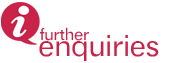﻿﻿﻿ Computational And Mathematical Biology (Requires Special Application) | INSTITUTE FOR MATHEMATICAL RESEARCH
» POSTGRADUATE » Fields of Study and Supervisors » Computational and Mathematical Biology (Requires special application)

# Computational and Mathematical Biology (Requires special application)

Computational and mathematical biology is an area that aims at the mathematical representation, analysis, and modeling of biological processes using a variety of applied mathematical techniques, such as calculus, probability theory, statistics, graph theory, topology, dynamical systems, differential equations, coding theory, and others. It has practical applications in biology, biotechnology, biomedical research and also epidemiology and population dynamics. Current research interest includes disease modeling, insilico modeling of proteins and bioinformatics.

List of SupervisorsAssoc. Prof. Dr. Shamarina ShohaimiTel : +603.9769.3177Email : shamarina@upm.edu.my

Updated:: 07/07/2021 [noorizzati]

MEDIA SHARING